# DAV Class 6 Maths Chapter 5 Worksheet 1 Solutions

The DAV Class 6 Maths Solutions and DAV Class 6 Maths Chapter 5 Worksheet 1 Solutions of Percentage and its Applications offer comprehensive answers to textbook questions.

## DAV Class 6 Maths Ch 5 WS 1 Solutions

Question 1.
What percent is
(a) ₹ 63 of ₹ 90
(b) 80 l of 240 l
(c) 16 of 250
(d) 200 cm of 1 metre
(e) 13 weeks of 1 year
(f) 1 dozen of 1 score.
Solution:
(a) ₹ 63 of ₹ 90
== 70%

(b) 80 l of 240 l
=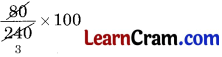= $$\frac{100}{3}$$ %
= 33$$\frac{1}{3}$$ %

(c) 16 of 250
=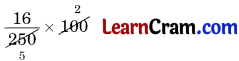= $$\frac{32}{5}$$
= 6.4 %

(d) 200 cm of 1 metre
=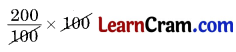= 200%

(e) 13 weeks of 1 year
= 13 weeks of 52 weeks
== 25%

(f) 1 dozen of 1 score
= 120 of 60
== 20 %Question 2.
There are 200 trees in an orchard. If 125 of them are apple trees, find the percentage of apple trees.
Solution:
Total number of trees = 200
Number of apple trees = 125
∴ Percentage of apple trees = $$\frac{125 \times 100}{200}$$
= $$\frac{125}{2}$$
= 62.5 %.

Question 3.
A man earns ₹ 12000 and spends ₹ 10500. If he saves rest of the money, find the percentage of his savings.
Solution:
Total money earned = ₹ 12000
Total money spent = ₹ 10,500
Money saved = ₹ 12000 – ₹ 10500 = ₹ 1500
∴ Percentage of saving =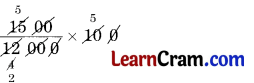= $$\frac{25}{2}$$ %
= 12 $$\frac{1}{2}$$ %Question 4.
There are 700 students in a school out of which 420 are girls. Find the percentage of boys in the school.
Solution:
Number of students in the school = 700
Number of girls = 420
∴ Number of boys = 700 – 420 = 280
∴ Percentage of boys == 40 %

Question 5.
Mansi obtained 410 marks out of 500 in her half yearly examination while Neha, her friend got 534 marks out of 600 marks. Find whose performance is better.
Solution:
Mansi got 410 marks out of 500
∴ her percentage of marks == 82%
Neha got 534 marks out of 600
∴ her percentage of marks =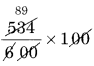= 89%
Hence Neha’s performance is better than Mansi.

Question 6.
What is the percentage of prime numbers from 1 to 10?
Solution:
Prime numbers between 1 and 10 are 2, 3, 5, 7 i.e. 4
∴ Percentage of prime numbers
= $$\frac{4}{10}$$ × 100
= 40%
Hence, the required percentage = 40%## DAV Class 6 Maths Chapter 5 Worksheet 1 Notes

Percent means per hundred. Its symbol is %. A fraction with denominator 100 is called percent.
e.g. $$\frac{17}{100}$$ = 17%,
$$\frac{8}{100}$$ = 8% and
$$\frac{120}{100}$$ = 120%

Example 1:
Express the following percents as decimals:

(a) 3.5 %
Solution:
3.5 % = $$\frac{3.5}{100}$$
= 0.035

(b) 2 %
Solution:
2 % = $$\frac{2}{100}$$
= 0.02

(c) 8.55 %
Solution:
8.55 % = $$\frac{8.55}{100}$$
= 0.0855

(d) 0.3 %
Solution:
0.3 % = $$\frac{0.3}{100}$$
= 0.03Example 2:
Write the following decimals as percents.

(a) 0.125
Solution:
0.125 × 100 = 12.5%

(b) 2.8
Solution:
2.8 × 100 = 280%

(c) 0.055
Solution:
0.055 × 100 = 5.5%

(d) 0.15
Solution:
0.15 × 100 = 15%

Example 3:
Convert the following ratios into Percents.

(a) 4 : 5
Solution:
4 : 5 =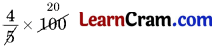= 80 %

(b) 6 : 10
Solution:
6 : 10 == 60 %

(c) 3 : 8
Solution:
3 : 8 == $$\frac{75}{2}$$
= 37.5 %

(d) 12 : 16
Solution:
12 : 16 == 75 %Example 4:
Write the mixed numbers as percent.

(a) 4 $$\frac{1}{2}$$
Solution:
4 $$\frac{1}{2}$$ == 450 %

(b) 3 $$\frac{1}{8}$$
Solution:
3 $$\frac{1}{8}$$ =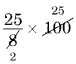= $$\frac{625}{2}$$
= 312.5 %

(c) 4 $$\frac{1}{4}$$
Solution:
4 $$\frac{1}{4}$$ =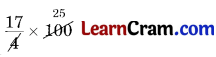= 425 %

(d) 6 $$\frac{1}{4}$$
Solution:
6 $$\frac{1}{4}$$ =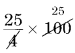= 625 %